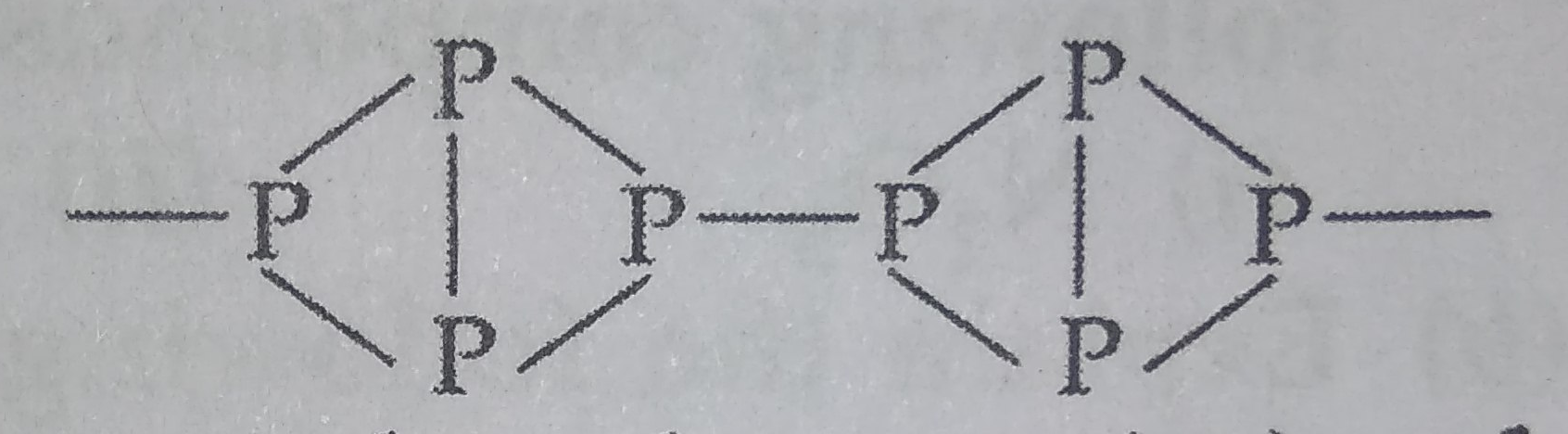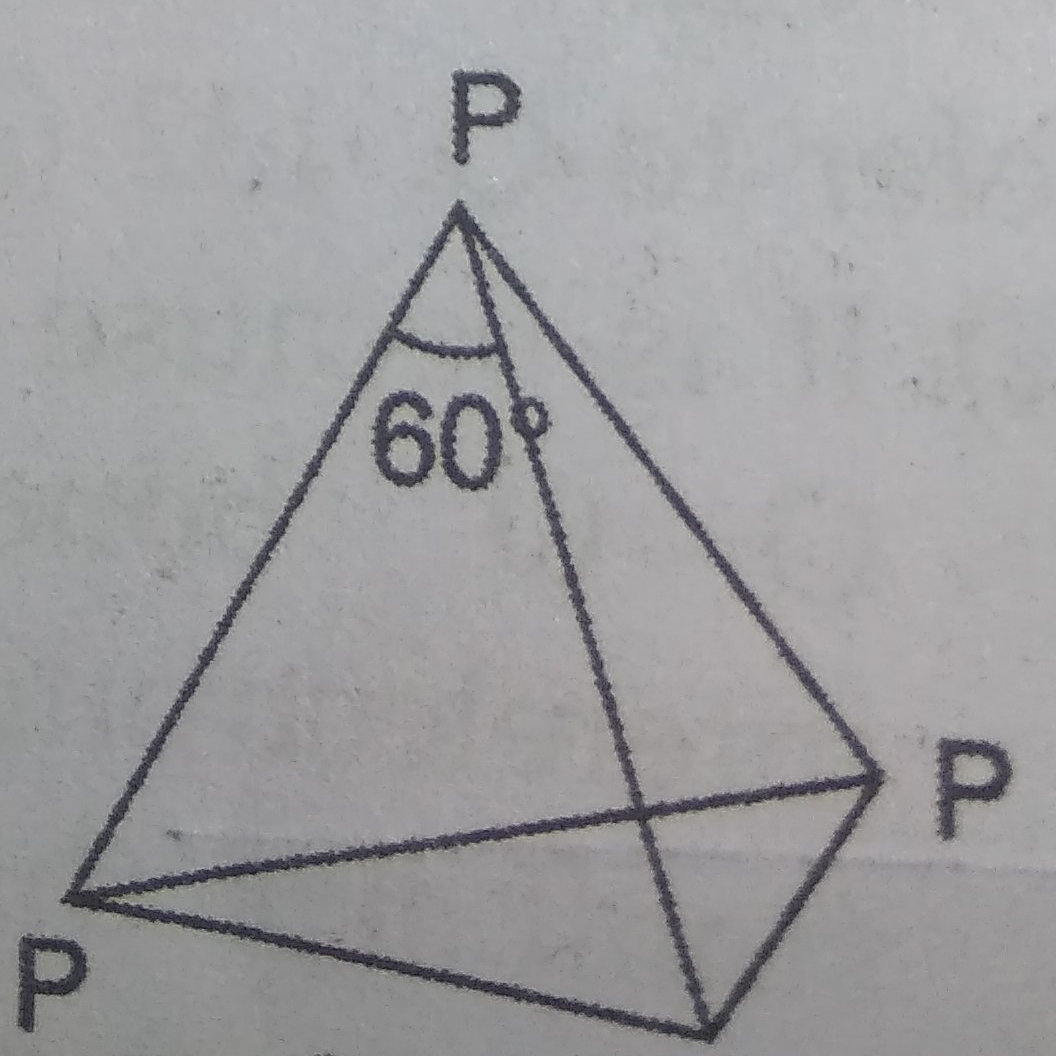# NEET Chemistry The p-Block Elements (XII) Questions Solved

BOARD

Account for the following :

(a) Thermal stability of water is much higher than that of ${H}_{2}S.$

(b) White phosphorus is more reactive than red phosphorus.

(c) Ammonia acts as a ligand.

(d) Bismuth is a strong oxidizing agent in pentavalent state.

(e) Concentrated sulphuric acid is a strong dehydrating agent.

(a) The thermal stability of hydrides decrease from ${H}_{2}O$ to ${H}_{2}S$ because as the size of the atom increases, the bond becomes weaker and thus breaks on heating.

(b) Red phosphorus is regarded as a polymer consisting of chains of ${P}_{4}$ tetrahedral linked together. This makes phosphorus denser and less reactiveBut in white phosphorus $\left({P}_{4}\right),$ the four phosphorus atoms lie at the corners of a regular tetrahedron. Each phosphorus atom is linked to each of the other three atoms by covalent bond. The bond angle is equal to $60°$ which suggests that the molecule is under strain and hence active in nature.(c) Due to the presence of a lone pair of electrons on nitrogen atom, it has a tendency to donate an electron pair, hence acts as a ligand.

(d) In Bismuth, the inert pair effect is very prominent. Thus +5 oxidation state is less stable in comparison to +3 oxidation state i.e. it readily accepts two electrons in pentavalent state and gets reduced to trivalent state. Therefore, it acts as a strong oxidising agent.

(e) Conc. ${H}_{2}S{O}_{4}$ has great affinity for water molecule i.e. it acts as a dehydrating agent.

Difficulty Level: